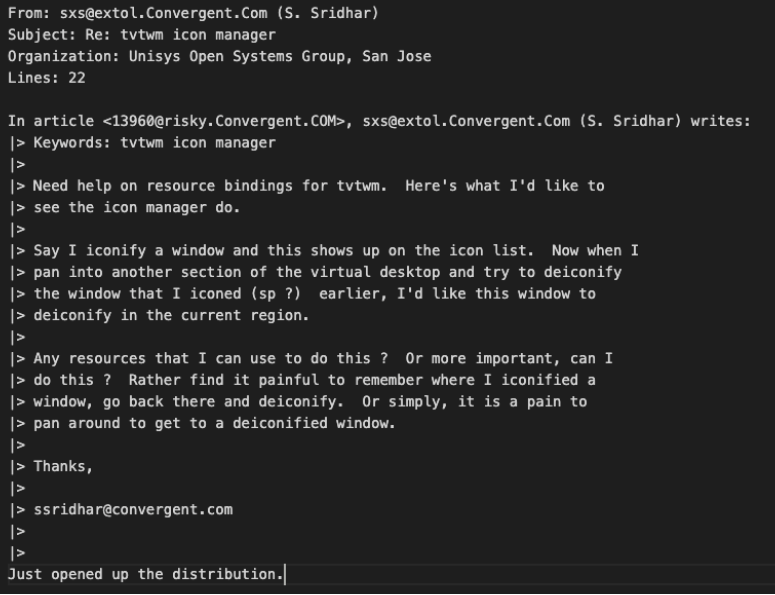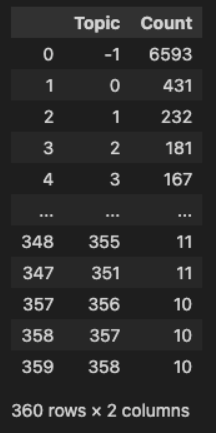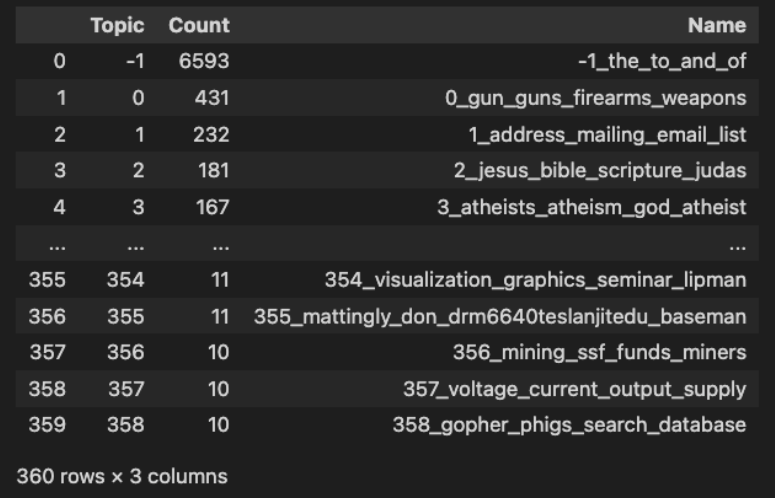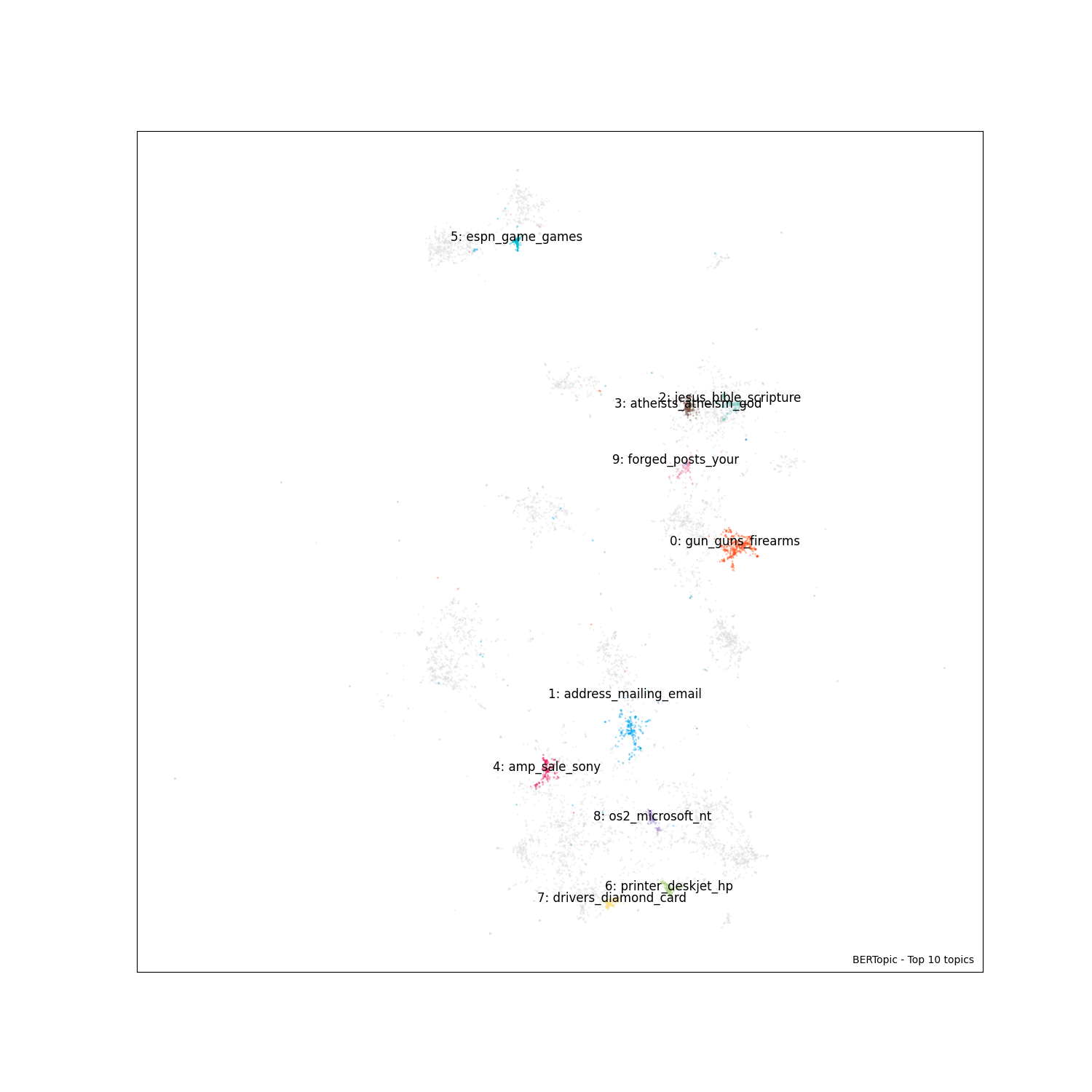All posts

# Python Topic Modeling With a BERT Model##### Share

Topic detection is a commonly sought-after Natural Language Processing (NLP) technique. It’s especially useful for getting high-level views of your conversations, emails, or documents. In this example, we’re going to take a look at BERT, a large language model, and how you can use a BERT-derived library to do topic detection.

## What is BERT?

BERT (“Bidirectional Encoder Representations from Transformers”) is a popular large language model created and published in 2018. BERT is widely used in research and production settings—Google even implements BERT in its search engine.

By 2020, BERT had become a standard benchmark for NLP applications with over 150 citations. At its core, it is built like many transformer models. The main difference between transformer models and Recurrent Neural Networks (RNNs), another classic in the NLP toolkit, is that they process the input all at once.

While RNNs have been around for decades, starting with the Hopfield network in the 1980s, RNNs evolved to include Long Short Term Memory (LSTM) Models by the end of the 1990s. More recent NLP architecture models hit the scene in the 2010s. In 2014, the Gated Recurrent Unit model was introduced to speed up LSTMs.

In 2017, transformer models were introduced. They not only allow for running predictions on the entire input at once but also more parallelization at training time. In the years since, transformer models are increasingly the architecture of choice for both NLP and image processing tasks.

The original BERT language model was trained on over 800 million words from BooksCorpus and over 2.5 billion words from Wikipedia. It was originally trained on two tasks: language modeling and next sentence prediction.

Since its inception, BERT has inspired many other models and use cases. One example is in topic detection with BERTopic, which we’ll cover below.

### Introduction to BERTopic

BERTopic is an open-source library that uses a BERT model to do Topic Detection with class-based TF-IDF procedure. TF-IDF stands for “Term Frequency - Inverse Document Frequency”. TF-IDF is an algorithm that weights the importance of words in a corpus, exactly as the name implies. The more frequently a word appears in a document, the more important it is. However, the more you see that word across documents, the less important it becomes.

An example of this could be the word “the”. You probably see the word “the” a lot in a single document. However, it also appears in many documents. On the other hand, a word like “BERT” would not appear in as many documents but may appear many times in a document on NLP. In this case, a TF-IDF model would probably say that BERT is an important word that defines the topic of a small set of documents.

## Using BERTopic for Topic Modeling in Python

Now that we’ve covered the basic history and ideas behind the BERT model and BERTopic library, let’s take a look at how we can use it. We’re not only going to use the library, but also explore the modeled data set, discuss the modeled topic, and visualize the resulting document clusters.

Before we get started, we’ll need to install a few libraries. We need BERTopic, Scikit Learn, NumPy, Pandas, and MatPlotLib. Install these libraries using the package manager of your choice. In this case, I’ll be using `pip install bertopic sklearn numpy pandas matplotlib`.

### Exploring a Topic Modeling Dataset

The first thing we’re going to do is get our BERTopic model and example dataset from `sklearn`

In this example, we use the set of documents stored in `fetch_20newsgroups` from the datasets in `sklearn`. All we need to do to get these documents is to call the `fetch_20newsgroups` function and extract the data element.

``````from bertopic import BERTopic
from sklearn.datasets import fetch_20newsgroups
# fetch an example dataset from sklearn
docs = fetch_20newsgroups(subset='all')['data']``````

Let’s take a brief look at the data we’re working with. The first thing we do is take a look at how much data we’re working with. In this case, we’ve extracted the data from the automatic return so we just call len on the list of documents.

``print(len(docs))``

The length of the document set is 18846 documents. Now that we know how long the dataset is, let’s look into it more. Let’s pull out two random elements from the dataset to see what each document looks like. For the next two examples, I’ve picked documents 1 and 100th (indexed at 0 and 44).

``print(docs)``Then, if we look at another email …

``print(docs)``From these couple of emails, we can see that the text data consists of a bunch of emails that are not all on the same topic. Once you have the list, feel free to explore it more if you’d like a better understanding of the data.

### This is Where the Magic Happens

Now that we’ve seen the dataset that we’re working with, it’s time to run topic detection on it. The nice thing about a library like BERTopic is the ability to do topic modeling in two lines of code. First, we initialize our model. Then, we use it to call `fit_transform` on the docs.

``````model = BERTopic()
topics, probs = model.fit_transform(docs)``````

### Understanding Modeled Topics

Now that we’ve modeled the topics in the dataset, let’s take a look at the model. We look at two things in this section, the topic frequency and the broader info about our topics. First, we look at the topic frequencies:

``model.get_topic_freq()``

We should see a result like the one below. The model shows us that it identified 359 topics. The topic marked “-1” is the set of topics that were too sparse to categorize. Interestingly, this is also the largest set of topics.We can get a little more information with the `get_topic_info` call.

``model.get_topic_info()``

As we can see in the image below, calling for topic info will also show us some of the top words in the topics. We can see some of the topics that the model picked out, including topics like: guns, emails, and Jesus.Visualizing BERTopic Modeled Topics

While it’s nice to be able to see the data in a chart, I thought it would be even nicer to see a visualization. In this code block below, we create a cluster graph to look at documents by topic. To do that, we’ll need `numpy`, `pandas`, `umap`, and `matplotlib`. (The `umap` library should have been automatically installed earlier as part of the package dependencies.)

After setting up our imports, we prep our data. We extract the model embeddings and fit them to a Uniform Manifold Approximation and Projection (UMAP) object, which contains the topic cluster data. Next, we put these cluster embeddings into a `pandas` dataframe along with the topics.

From there, we define some plot parameters for our visualization. In this case, the number of topics we want to see and the font size. Next, we slice the data up so we only plot the top 10 topics and create a colormap which `matplotlib` will use to color our graph.

Now that we’ve configured the visualization, it’s time to make the plot. We use `matplotlib` to create a scatter plot. The scatter plot we create in this example plots the outliers in gray and the top 10 topics as colored clusters. Then, we add the cluster centroid titles to the scatter plot, and voila.

``````import numpy as np
import pandas as pd
from umap import UMAP

import matplotlib
import matplotlib.pyplot as plt

%matplotlib inline

# Prepare data for plotting
embeddings = model._extract_embeddings(docs, method="document")
umap_model = UMAP(n_neighbors=10, n_components=2, min_dist=0.0, metric='cosine').fit(embeddings)
df = pd.DataFrame(umap_model.embedding_, columns=["x", "y"])
df["topic"] = topics

# Plot parameters
top_n = 10
fontsize = 12

# Slice data
to_plot = df.copy()
to_plot[df.topic >= top_n] = -1
outliers = to_plot.loc[to_plot.topic == -1]
non_outliers = to_plot.loc[to_plot.topic != -1]

# Visualize topics
cmap = matplotlib.colors.ListedColormap(['#FF5722', # Red
'#03A9F4', # Blue
'#4CAF50', # Green
'#80CBC4', # FFEB3B
'#673AB7', # Purple
'#795548', # Brown
'#E91E63', # Pink
'#212121', # Black
'#00BCD4', # Light Blue
'#CDDC39', # Yellow/Red
'#AED581', # Light Green
'#FFE082', # Light Orange
'#BCAAA4', # Light Brown
'#B39DDB', # Light Purple
'#F48FB1', # Light Pink
])

# Visualize outliers + inliers
fig, ax = plt.subplots(figsize=(15, 15))
scatter_outliers = ax.scatter(outliers['x'], outliers['y'], c="#E0E0E0", s=1, alpha=.3)
scatter = ax.scatter(non_outliers['x'], non_outliers['y'], c=non_outliers['topic'], s=1, alpha=.3, cmap=cmap)

# Add topic names to clusters
centroids = to_plot.groupby("topic").mean().reset_index().iloc[1:]
for row in centroids.iterrows():
topic = int(row.topic)
text = f"{topic}: " + "_".join([x for x in model.get_topic(topic)[:3]])
ax.text(row.x, row.y*1.01, text, fontsize=fontsize, horizontalalignment='center')

ax.text(0.99, 0.01, f"BERTopic - Top {top_n} topics", transform=ax.transAxes, horizontalalignment="right", color="black")
plt.xticks([], [])
plt.yticks([], [])
plt.savefig("BERTopic_Example_Cluster_Plot.png")
plt.show()``````

The image below shows the example cluster plot created from the document set we used. You probably want to click on the image for a closer look. Or, just create it yourself from the code above!## Summary of BERT Topic Modeling in Python

In this post, we covered how to get a topic model from a BERT model in Python. We started by taking a look into what BERT and BERTopic are. Then, we used the BERTopic Python library and Scikit Learn to see what a topic model does and looks like.

We fit the BERTopic model to a dataset of almost 20k emails from `sklearn`. Once we had the model predictions, we looked at what was modeled, and proceeded to make a visualization. We used matplotlib and UMAP to create a nice scatter plot to visualize the top 10 topics in our data. Check out the code on GitHub and join the discussion!

If you have any feedback about this post, or anything else around Deepgram, we'd love to hear from you. Please let us know in our GitHub discussions.